# Chapter 17 Electric Potential Electric Energy and Capacitance

• Slides: 20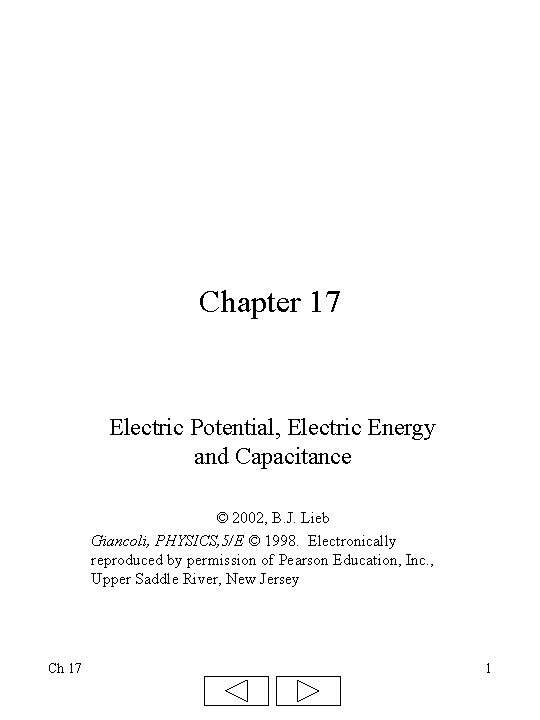Chapter 17 Electric Potential, Electric Energy and Capacitance © 2002, B. J. Lieb Giancoli, PHYSICS, 5/E © 1998. Electronically reproduced by permission of Pearson Education, Inc. , Upper Saddle River, New Jersey Ch 17 1Review of Work and Energy Electric potential is based on the concept of work and energy. • Work = (force) (distance) (cos ) • Units are J (joules) 1 J = N m • Potential Energy is energy of position such as the energy stored in a stretched spring or a roller coaster at the top of the first hill • You have to do work to move a positive charge in an electric field to point a, so that charge has electrical potential energy (Pea) • If you release the charge it will “fall” away from the other charge thus gaining kinetic energy KE = ½ m v 2 Ch 17 2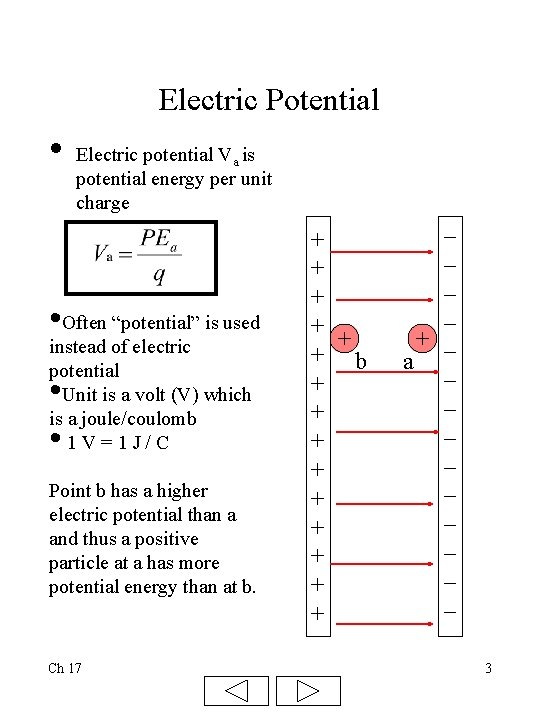Electric Potential • Electric potential Va is potential energy per unit charge • Often “potential” is used instead of electric potential Unit is a volt (V) which is a joule/coulomb 1 V=1 J/C • • Point b has a higher electric potential than a and thus a positive particle at a has more potential energy than at b. Ch 17 + + + b + + + + + _ _ + _ a _ _ _ _ _ 3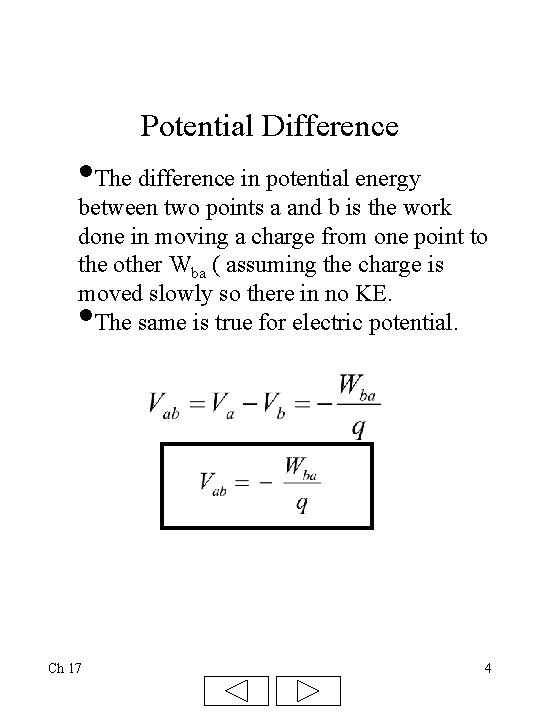Potential Difference • The difference in potential energy between two points a and b is the work done in moving a charge from one point to the other Wba ( assuming the charge is moved slowly so there in no KE. The same is true for electric potential. • Ch 17 4Potential Energy Difference • Since electric potential is potential energy per unit charge, we can find the change in potential energy Example: The two parallel plates shown are connected to a 9 V battery. If the charge is +4 C, then the potential PE = (9 V) (4 C)= 36 J If the charge were released at point b it would “fall” to a and gain 36 J of kinetic energy if there was no friction. • Ch 17 + + + b + + + + + _ _ + _ a _ _ _ _ _ 5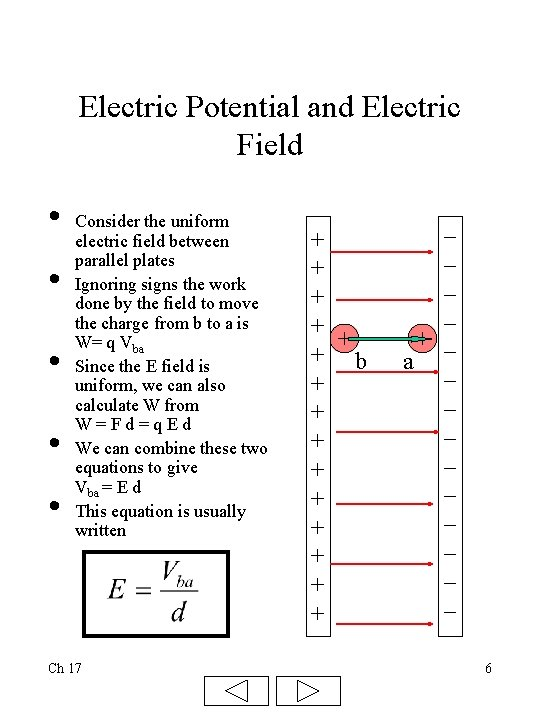Electric Potential and Electric Field • • • Consider the uniform electric field between parallel plates Ignoring signs the work done by the field to move the charge from b to a is W= q Vba Since the E field is uniform, we can also calculate W from W=Fd=q. Ed We can combine these two equations to give Vba = E d This equation is usually written Ch 17 + + + b + + + + + _ _ + _ a _ _ _ _ _ 6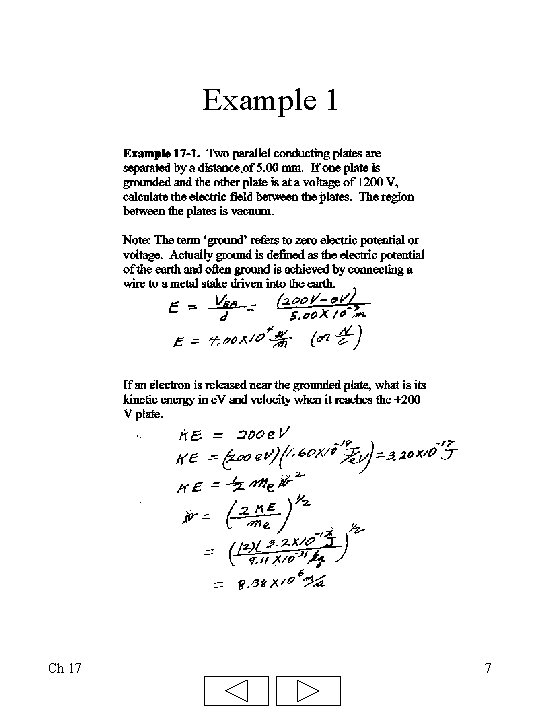Example 1 Ch 17 7Equipotential Lines • • • A 9 volt battery is connected to the parallel plates Thus the positive plate is at a potential of 9 V and the negative plate is a 0 V. The green lines are called equipotential lines because every point on the line is at the same electrical potential Notice how the voltage drops as you go from the 9 V plate, which is also an equipotential to the negative plate at 0 V The green lines are actually surfaces in 3 D The equipotentials are always perpendicular to the electric field lines. 9 volts 7. 75 V Ch 17 _ _ _ _ + + + + 4. 5 V 2. 25 V 8Equipotential Lines of Two Point Charges Ch 17 9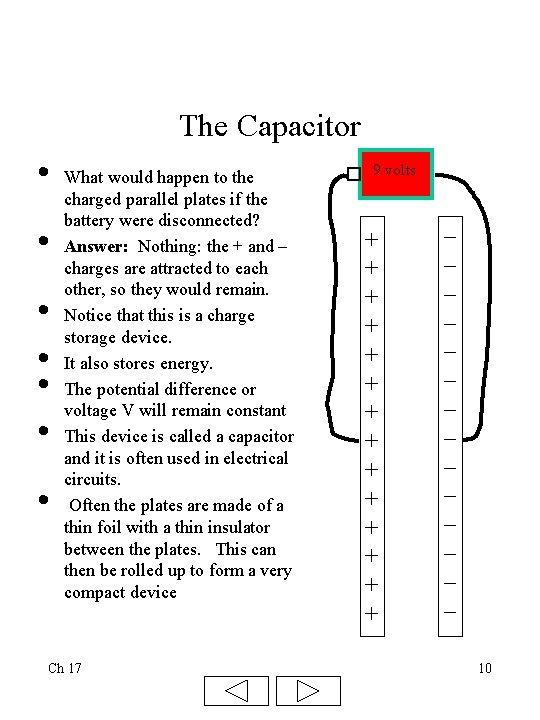The Capacitor • • What would happen to the charged parallel plates if the battery were disconnected? Answer: Nothing: the + and – charges are attracted to each other, so they would remain. Notice that this is a charge storage device. It also stores energy. The potential difference or voltage V will remain constant This device is called a capacitor and it is often used in electrical circuits. Often the plates are made of a thin foil with a thin insulator between the plates. This can then be rolled up to form a very compact device Ch 17 9 volts + + + + _ _ _ _ 10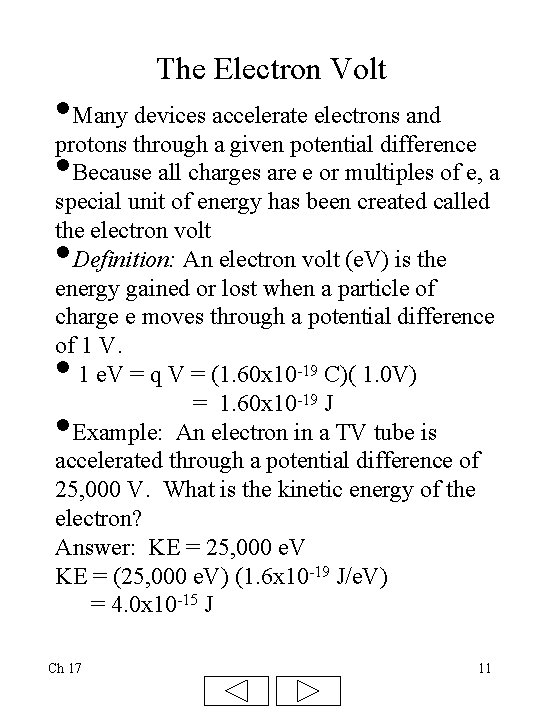The Electron Volt • Many devices accelerate electrons and protons through a given potential difference • Because all charges are e or multiples of e, a special unit of energy has been created called the electron volt Definition: An electron volt (e. V) is the energy gained or lost when a particle of charge e moves through a potential difference of 1 V. 1 e. V = q V = (1. 60 x 10 -19 C)( 1. 0 V) = 1. 60 x 10 -19 J Example: An electron in a TV tube is accelerated through a potential difference of 25, 000 V. What is the kinetic energy of the electron? Answer: KE = 25, 000 e. V KE = (25, 000 e. V) (1. 6 x 10 -19 J/e. V) = 4. 0 x 10 -15 J • • • Ch 17 11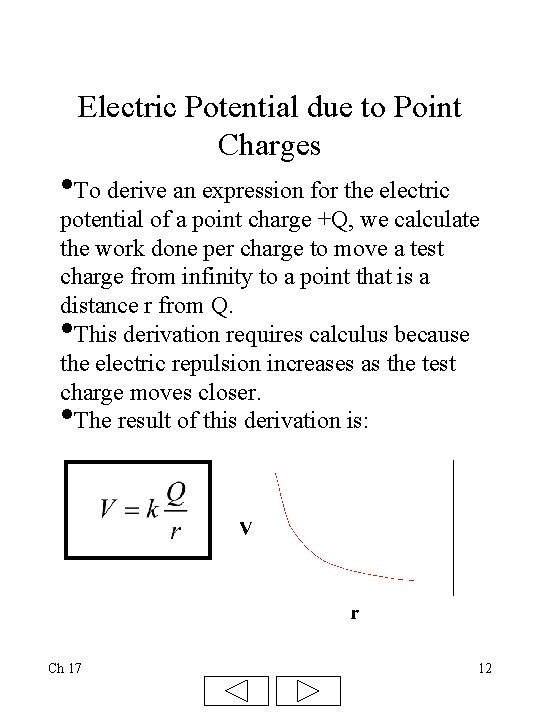Electric Potential due to Point Charges • To derive an expression for the electric potential of a point charge +Q, we calculate the work done per charge to move a test charge from infinity to a point that is a distance r from Q. This derivation requires calculus because the electric repulsion increases as the test charge moves closer. The result of this derivation is: • • Ch 17 12Example 2 Ch 17 13Capacitor Equations I We will now derive several equations that describe the capacitor. • The unit of capacitance is the farad (F). • Q is the charge on one plate (and there is an equal but opposite charge on the other plate) and V is the voltage. • 1 F=1 C/V • We refer to C as the capacitance. • Most capacitors are F or smaller Ch 17 + + + + _ _ _ _ 14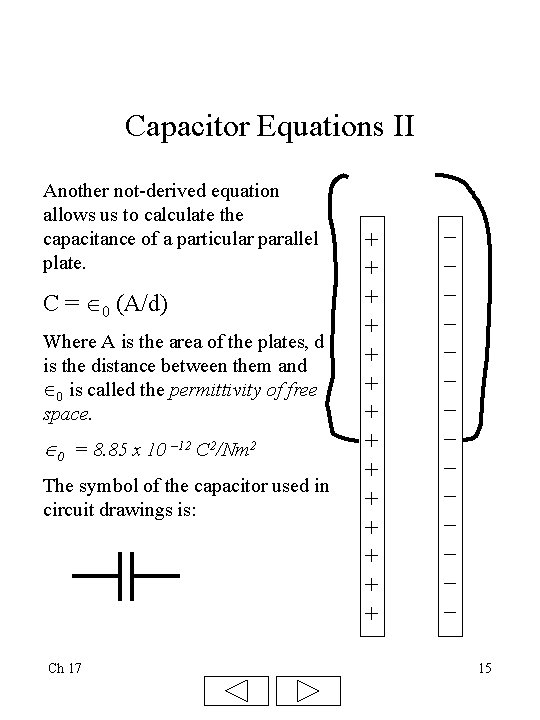Capacitor Equations II Another not-derived equation allows us to calculate the capacitance of a particular parallel plate. C = 0 (A/d) Where A is the area of the plates, d is the distance between them and 0 is called the permittivity of free space. 0 = 8. 85 x 10 – 12 C 2/Nm 2 The symbol of the capacitor used in circuit drawings is: Ch 17 + + + + _ _ _ _ 15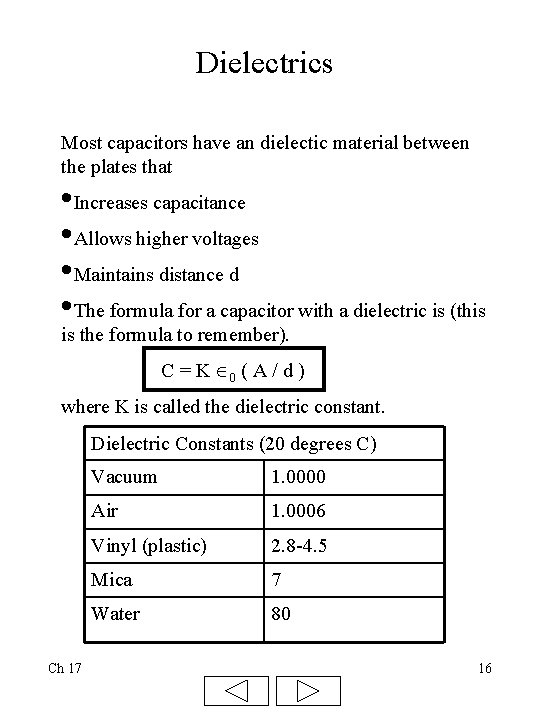Dielectrics Most capacitors have an dielectic material between the plates that • Increases capacitance • Allows higher voltages • Maintains distance d • The formula for a capacitor with a dielectric is (this is the formula to remember). C = K 0 ( A / d ) where K is called the dielectric constant. Dielectric Constants (20 degrees C) Ch 17 Vacuum 1. 0000 Air 1. 0006 Vinyl (plastic) 2. 8 -4. 5 Mica 7 Water 80 16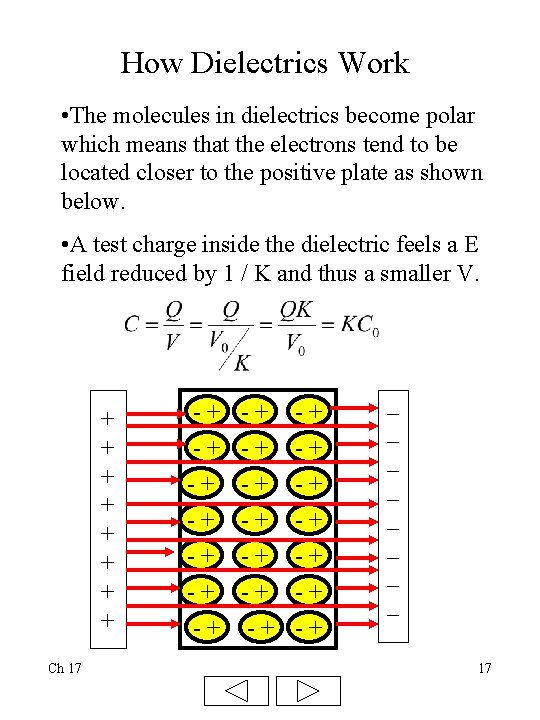How Dielectrics Work • The molecules in dielectrics become polar which means that the electrons tend to be located closer to the positive plate as shown below. • A test charge inside the dielectric feels a E field reduced by 1 / K and thus a smaller V. + + + + Ch 17 -+ -+ -+ -+ -+ -+ _ _ _ _ 17Energy Storage in a Capacitor A battery must do work to move electrons from one plate to the other. The work done to move a small charge q across a voltage V is W = V q. As the charge increases, V increases so the work to bring q increases. Using calculus we find that the energy (U) stored on a capacitor is given by: Ch 17 18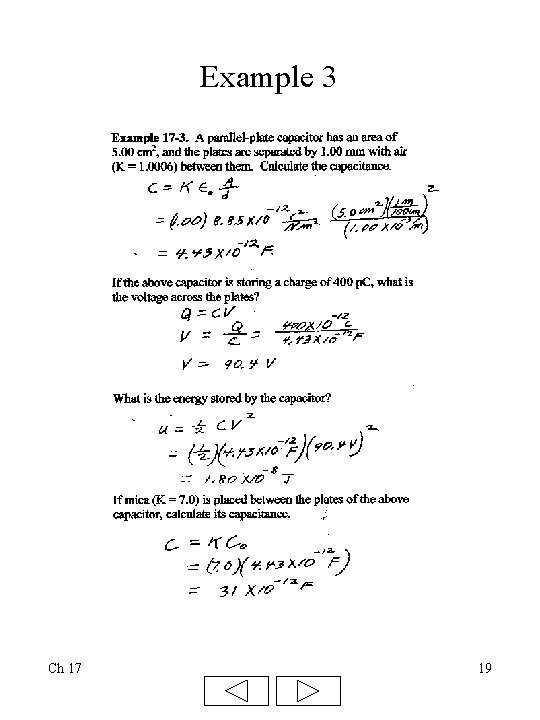Example 3 Ch 17 19Cathode Ray Tube (CRT) • Electrons are ejected from heated metal called cathode • Then accelerated through potential difference of as much as 25, 000 V • Electron beam is then bent by horizontal and vertical electric fields created by the horizontal and vertical deflection plates. • Electron beam then strikes fluorescent screen • Points on screen fluoresce in different colors • Electronics attached to deflection plates cause beam to paint a picture on screen • Picture made with 525 horizontal lines • Picture redrawn at 30 Hz Ch 17 20